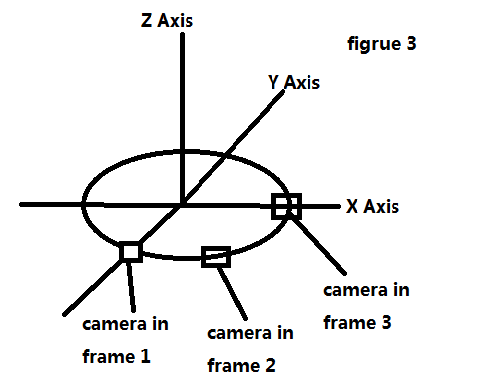# Panda3D Hello World Tutorial Questions

Hi,
I’m reading Panda3D “Hello World Tutorial”, and I have several questions:
1.

My mouse has only left and right button, but has no middle button. How can I simulate these two middle button operations?
2. What is the coordinate that Panda3D uses? Do I draw right (figure 1)?
3.

``````def spinCameraTask(self, task):
angleRadians = angleDegrees * (pi / 180.0)
self.camera.setHpr(angleDegrees, 0, 0)

What is the type of variable “task”, and what is the meaning of the “task.time”?
4.

``   self.camera.setPos(20 * sin(angleRadians), -20.0 * cos(angleRadians), 3)``

Why we need to compute the camera’s x and y coordinates as this? I understand that z = 3 as to move the camera up a bit along the vertical axis.
5. What the default position of the camera, like this (figure 2)?
6.

`````` self.camera.setPos(20 * sin(angleRadians), -20.0 * cos(angleRadians), 3)
self.camera.setHpr(angleDegrees, 0, 0)``````

Does the order of “setPos” and “setHpr” matter? For example, first translation, second rotation is different as first rotation, second translation in OpenGL.
7. I don’t understand this tutorial well, and I want to know where is the camera’s position in every frame, and the direction it look at (figure 3). May someone explain it in more detail?
Thanks!1. Sorry, dont know. In your apps you’ll probably just use other keys for this, but might it be an option to buy a mouse with mouse button?2. Panda uses a Z-up right handed coordinate system, so your drawing is correct.

``self.taskMgr.add(self.spinCameraTask,"SpinCameraTask")``

1. So this function is run every frame (which can be seen from the return-statement, which says ‘return Task.cont’, instead of for intance ‘Task.done’), and the task variable is accessed to find out how long this task has been executed at each specific frame. To make the camera rotate around the scene (which is the anwser to your last question), it has to be undated to jump to a new position every frame. This new position is determined by increasing the angle at which the camera is located wrt the origin with time
``````angleDegrees = task.time*6.0